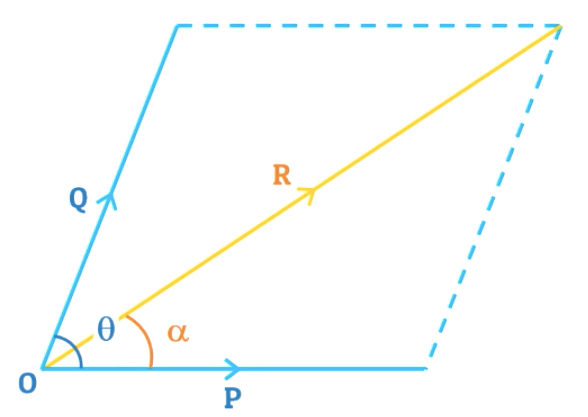# Law of Parallelogram of Forces : 5 in 5 MCQs S01-E01

As solved in the video under 5 minutes, this is the notes for the 5 MCQs solved with explanation. MCQ type questions are not only helpful for your examinations but also for competitive exams like GATE, UPSC and many more.

# What is Law of Parallelogram of Forces?Consider two forces $P$ and $Q$ acting simultaneously on a particle $O$ as shown in the figure above. Let the angle between these two forces be $\theta$. By extending imaginary lines from $P$ and $Q$, we can get a parallelogram as shown in the figure above as dotted lines.

Therefore, by the Law of Parallelogram of Forces the resultant of the forces $P$ and $Q$ can be represented by the diagonal of this parallelogram and its direction as $\alpha \degree$ from force $P$.

# Definition of Law of Parallelogram of Forces

If two forces acting simultaneously on a particle are represented in magnitude and direction by the two sides of a parallelogram, then their resultant can be represented in magnitude and direction by the diagonal of the parallelogram which passes through their point of intersection.

And the magnitude of the resultant is given by:

$R = \sqrt{P^2 + Q^2 + 2 \ PQ \ \cos \theta}$

And the direction of the resultant is given by:

$\tan \alpha = {Q \ \sin \theta \over P + Q \ \cos \theta}$

Now, let's start solving MCQs. These questions will have 2 forces acting on a particle, hence we will apply the law of parallelogram of forces.

## If $P \gt Q$, and $P$ and $Q$ are acting along a same straight line, but in opposite direction. Then the resultant is?

a) $P+Q$              c) $P \over Q$

b) $P-Q$              d) $Q \over P$

Given:
$\theta = 180 \degree$ (because P and Q are in straight line, but opposite in direction)

\begin{aligned} R &= \sqrt{P^2 + Q^2 + 2 \ PQ \ \cos \theta} \\ &= \sqrt{P^2 + Q^2 + 2 \ PQ \ \cos 180} \\ &= \sqrt{P^2 + Q^2 - 2 \ PQ} \\ &= \sqrt{(P - Q)^2} \\ & \boxed{\therefore R = P - Q} \\ \end{aligned}## The resultant of two equal forces P making an angle of $\theta$ is:

a) $2P \sin {\theta \over 2}$              c) $2P \tan {\theta \over 2}$

b) $2P \cos {\theta \over 2}$              d) $2P \cot {\theta \over 2}$

Given:
$P = Q$

\begin{aligned} R &= \sqrt{P^2 + Q^2 + 2 \ PQ \ \cos \theta} \\ &= \sqrt{P^2 + P^2 + 2 \ P \sdot P \ \cos \theta} \\ &= \sqrt{2P^2 + 2 \ P^2 \cos \theta} \\ &= \sqrt{2P^2 (1 + \cos \theta)} \\ &= \sqrt{2P^2 (2 \cos^2 {\theta \over 2})} \\ &\boxed{\therefore R = 2P \cos {\theta \over 2}} \end{aligned}

## The angle between two forces when resultant is maximum and minimum respectively is:

a) $0 \degree \And 180 \degree$              c) $90 \degree \And 180 \degree$

b) $180 \degree \And 0 \degree$              d) $90 \degree \And 0 \degree$

Explanation:
In the question it is asked to find the value of $\theta$ for the maximum value $R$ and the minimum value of $R$.

We know that: $R = \sqrt{P^2 + Q^2 + 2 \ PQ \ \cos \theta}$.
Now if we change the value of $cos \theta$ and add or subtract it from the remaining value of $P$ and $Q$ we get the value of $R$.

So, to find the answer, let's take 4 extreme values from the unit circle as given in the options for $\theta \to 0 \degree, \ 90 \degree, \ 180 \degree \ and \ 270 \degree$
Now the $\cos$ values for these angles will be $\to 1, 0, -1 \ and \ 0$ respectively.

Here, maximum value is $1$(i.e. $\cos 0$) and minimum value is $-1$(i.e. $\cos 180$).
Therefore, at $0 \degree$ and at $180 \degree$, the resultant will be maximum and minimum respectively.

## The resultant of two forces each equal to P and acting at right angle is:

a) ${P \over 2}$              c) ${P \over 2 \sqrt2}$

b) ${P \over \sqrt2}$              d) $\sqrt{2} \sdot P$

Given:
$P = Q$, $\theta = 90\degree$

\begin{aligned} R &= \sqrt{P^2 + Q^2 + 2 \ PQ \ \cos \theta} \\ &= \sqrt{P^2 + Q^2 + 2 \ PQ \ \cos 90} \\ &= \sqrt{P^2 + P^2} \\ &= \sqrt{2P^2} \\ &\boxed{R = \sqrt{2} \sdot P} \\ \end{aligned}

## The resultant of two forces $P$ and $Q$ is $R$. If $Q$ is doubled, the new resultant is perpendicular to P. Then,

a) ${P = Q}$              c) ${Q = R}$

b) ${Q = 2R}$              d) $\text{none of these}$

Explanation:
We have two set of data here:

1. Forces $P$ and $Q$, whose resultant is R
2. If $Q$ is doubled, then resultant(R') would be perpendicular to $P$. This means $\alpha = 90 \degree$ and $Q = 2Q$
\begin{aligned} For \ \alpha &= 90 \degree, \ Q = 2Q \ \text{we know that} \\ \\ \tan \alpha &= {Q \ \sin \theta \over P + Q \ \cos \theta} \\ \\ \therefore \tan 90 &= {2Q \sin \theta \over P + Q \ \cos \theta} \\ \\ \therefore \infin &= {2Q \sin \theta \over P + Q \ \cos \theta} \\ \end{aligned}

This is only possible if:

\begin{aligned} &P + Q \ \cos \theta = 0 \\ &\boxed{\therefore P = -2Q \cos \theta} ---- (1) \end{aligned}

Using the formula for resultant force:

\begin{aligned} R &= \sqrt{P^2 + Q^2 + 2 \ PQ \ \cos \theta} \\ &\text{Using from equation (1)} \\ &= \sqrt{(-2Q \cos \theta)^2 + Q^2 + 2(-2Q \cos \theta) \sdot Q cos \theta} \\ &= \sqrt{4Q^2 \cos^2 \theta + Q^2 - 4Q^2 \cos^2 \theta} \\ &= \sqrt{Q^2} \\ &\boxed{\therefore R = Q} \end{aligned}